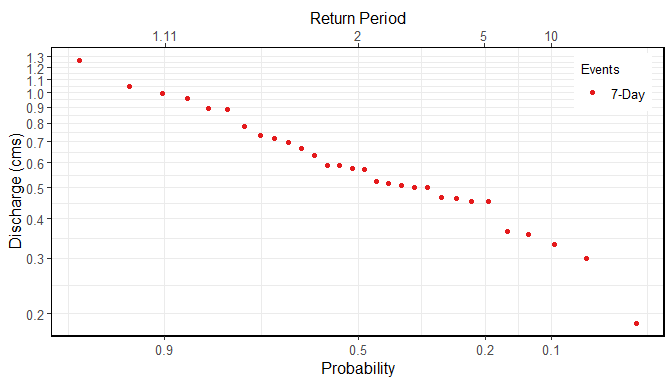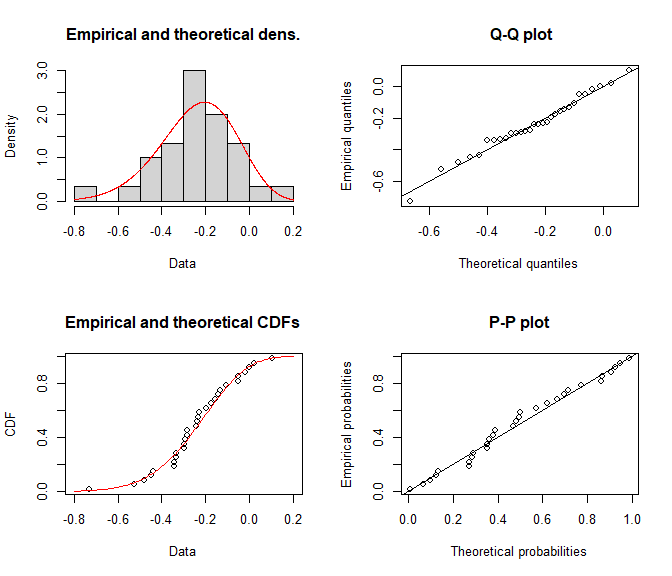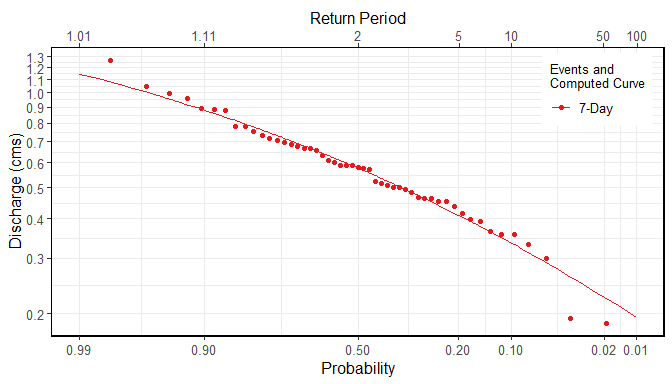Computing Frequency Analyses with fasstr

fasstr, the Flow Analysis Summary Statistics Tool for R, is a set of R functions to tidy, summarize, analyze, trend, and visualize streamflow data. This package summarizes continuous daily mean streamflow data into various daily, monthly, annual, and long-term statistics, completes trending and frequency analyses, with outputs in both table and plot formats.

This vignette is a guide on the various volume frequency analysis functions found in fasstr to plot frequency data and determine frequency quantile statistics using the Log-Pearson Type III or Weibull distributions. In this vignette you’ll learn what functions to use for specific analyses, arguments to customize analyses, and what results and outputs are produced.

Overview

Computing a frequency analysis with fasstr allows for options and customization of both the inputs and outputs. These functions plot probabilities of flow data using chosen plotting options and calculates frequency quantiles (ex. 7Q10) based on fitting data to either Log-Pearson Type III or Weibull distributions. There are four functions within fasstr that conduct frequency analyses:

1. compute_annual_frequencies() - conduct an annual frequency analysis from daily streamflow data (analysis calculates minimums/maximums and subsequently ranks that data).
2. compute_frequency_quantile() - conduct an annual frequency analysis from daily streamflow data and return a specific quantile based on a duration (rolling mean days) and return period (analysis calculates minimums/maximums and subsequently ranks that data).
3. compute_HYDAT_peak_frequencies() - conduct an annual frequency analysis from instantaneous peak data (minimum or maximum) for stations from a HYDAT database.
4. compute_frequency_analysis() - conduct a frequency analysis with custom data (analysis ranks data provided; is the main frequency analysis function used within each of the frequency analysis functions).

With the exception of the compute_frequency_quantile() function which only produces a quantile value, the frequency functions produce five outputs contained within a list. See the sections below for more information on each output and options. The five outputs include the following:

1. Freq_Analysis_Data - data used for analysis (either data provided in compute_frequency_analysis(), computed extremes in compute_annual_frequencies(), or HYDAT extracted extremes in compute_HYDAT_peak_frequencies()).
2. Freq_Plot_Data - data used to plot probabilities based on the selected plotting positions (weibull, hazen, or median), includes the event values, probability and return periods. Option to include/exclude the compute curve.
3. Freq_Plot - plot of event probabilities based on the selected plotting positions (weibull, hazen, or median).
4. Freq_Fitting - a fitdistplus::fitdist object that contains information on the computed curve based on the selected distribution (Pearson Type III (or log of) or weibull) and fitting methods (methods of moments or maximum likelihood estimation).
5. Freq_Fitted_Quantiles - the computed fitted quantiles based on the selected fitting options and selected quantile probabilities.

Functions and Data Inputs

Annual Daily Minimums/Maximums

• compute_annual_frequencies()
• compute_frequency_quantile()

To determine frequencies of annual daily minimum or daily maximum flows, or of any duration days, from a daily streamflow data set, the compute_annual_frequencies() and compute_frequency_quantile() functions will take daily data, either from HYDAT using the station_number argument or your own data frame of data using the data argument to complete an analysis. As with most fasstr functions, options for rolling days, missing values, and date filtering can be completed using the function arguments (roll_days, water_year_start, etc).

The compute_annual_frequencies() function will produce all five outputs from the analysis, as listed above, including the plotting data, plot, and computed quantiles. If just the quantile is desired, and assuming your data fits the selected distributions, the compute_frequency_quantile() function can be used. By supplying the desired duration (roll_days argument) and the desired return period (return_period argument) a single numeric quantile value will be returned from the data.

Annual Peak Minimums/Maximums from HYDAT

• compute_HYDAT_peak_frequencies()

To determine frequencies of annual instantaneous minimum or maximum flows from stations from HYDAT, the compute_HYDAT_peak_frequencies() function will extract the data, if available, and complete the analysis. As this data is extracted from HYDAT by listing the station using the station_number argument and no pre-filtering is completed on the data, the data argument and many of the filtering arguments are not available for this function. If you have a data frame of your own instantaneous maximums or minimums, a custom analysis can be completed using the compute_frequency_analysis() function as described below.

Custom Data

• compute_frequency_analysis()

To complete a frequency analysis using custom data, like peaks-over-threshold analyses, the compute_frequency_analysis() function will take the provided data and complete the analysis. The data provided must contain three columns:

1. events - the year or other label to identify the specific flow event (could have 1999a and 1999b if doing a peaks-over-threshold).
2. values - the flow value in cubic metres per second.
3. measures - the type of flow value/event (i.e. “Inst. Peak” or “7-Day Low”); can have multiple measures (ex. 3-day and 7-day) that will be plotted and computed.

Here is an example of data the can be provided, wrangled from the annual lowflows function:

low_flows <- calc_annual_lowflows(station_number = "08NM116",
start_year = 1980,
end_year = 2000,
roll_days = 7)
low_flows <- dplyr::select(low_flows, Year, Value = Min_7_Day)
low_flows <- dplyr::mutate(low_flows, Measure = "7-Day")
low_flows
# A tibble: 21 x 3
Year Value Measure
<dbl> <dbl> <chr>
1  1980 0.655 7-Day
2  1981 0.885 7-Day
3  1982 1.05  7-Day
4  1983 0.634 7-Day
5  1984 0.784 7-Day
6  1985 0.589 7-Day
7  1986 0.719 7-Day
8  1987 0.355 7-Day
9  1988 0.186 7-Day
10  1989 0.577 7-Day
# ... with 11 more rows

This data can then be applied to the compute_frequency_analysis() function. This example has the default column names in the data and this do not need to be listed, but are shown for demonstration.

compute_frequency_analysis(data = low_flows,
events = Year,
values = Value,
measures = Measure)

Usage, Options, and Outputs

Analysis Data

The returned Freq_Analysis_Data object provides the raw data used in the frequency analyses. Based on the selected frequency function used, this tibble will contain the respective data; the provided values with the custom analysis, the computed extremes with the annual analysis, and the HYDAT extreme peaks from HYDAT in the peak analysis. See each functions’ documentation for more information. This tibble object provides the analysis data as the annual and HYDAT peak data are calculated or extracted from HYDAT.

To provide examples of the outputs, an annual analysis will be completed on a Mission Creek HYDAT station (the plot_curve argument is set to FALSE for the start of this example):

freq_analysis <- compute_annual_frequencies(station_number = "08NM116",
start_year = 1981,
end_year = 2010,
roll_days = 7,
plot_curve = FALSE)

The following is an example of the returned Freq_Analysis_Data tibble:

freq_analysis$Freq_Analysis_Data # A tibble: 30 x 3 Year Measure Value <dbl> <fct> <dbl> 1 1981 7-Day 0.885 2 1982 7-Day 1.05 3 1983 7-Day 0.634 4 1984 7-Day 0.784 5 1985 7-Day 0.589 6 1986 7-Day 0.719 7 1987 7-Day 0.355 8 1988 7-Day 0.186 9 1989 7-Day 0.577 10 1990 7-Day 0.958 # ... with 20 more rows Frequency Plotting Based on the analysis data in the Freq_Analysis_Data object, the data is ranked, by default for low-flow frequencies, from low to high with the lowest flow value ranked at 1. To complete high-flow analyses and rank the data from high to low, set the use_max argument to TRUE. The probabilities of each event are then determined using the following generalize plotting equation: • P = (m - A) / (n + 1 - A - B) where: • m is the rank of the value • n the total number of events in the data • A & B are the constants depending on which plotting position is used (weibull, median, or hazen) The probability plotting positions (A and B constants) are selected using the prob_plot_position argument, listing 'weibull' where A and B are 0, 'median' where A and B are 0.3, or 'hazen' where A and B are 0.5. The selected plotting position does not have an effect on the final computed curve. To plot the data on a logarithmic scale, set the use_log argument to TRUE. With these options set, the data used for plotting is returned in the Freq_Plot_Data tibble object. The events are sorted by measure, and ranked by the event value, and provides the probability and the return period for each event, used for plotting. See the following for an example of this output: freq_analysis$Freq_Plot_Data
# A tibble: 30 x 5
Year Measure Value Probability Return Period
<dbl> <fct>   <dbl>       <dbl>           <dbl>
1  1988 7-Day   0.186      0.0323           31
2  1993 7-Day   0.298      0.0645           15.5
3  2002 7-Day   0.331      0.0968           10.3
4  1987 7-Day   0.355      0.129             7.75
5  2003 7-Day   0.363      0.161             6.2
6  2010 7-Day   0.454      0.194             5.17
7  2007 7-Day   0.454      0.226             4.43
8  2008 7-Day   0.463      0.258             3.88
9  1994 7-Day   0.465      0.290             3.44
10  2009 7-Day   0.502      0.323             3.1
# ... with 20 more rows

This data is then used for plotting and returned in the Freq_Plot ggplot2 object. See the example below. To change the probabilities/vertical lines shown on the x-axis, change the values using the prob_scale_points argument to list the breaks.

freq_analysis$Freq_PlotDistribution Fitting and Computing Frequency Quantiles The fasstr functions also compute frequency quantiles, like commonly used 7Q5, 7Q10, 5Q30, etc. Calculating frequency quantiles requires fitting historical event data (annual minimums, maximums or others) to a probability distribution (i.e. Log-Pearson Type III or Weibull in fasstr). The flow quantiles are then extracted from the distribution for given probabilities (and equivalent return periods). In the fasstr frequency analysis functions, this is done so by choosing a probability distribution and method of fitting to fit the data (may require data exploration for determining most appropriate distribution). Results from this fitting are found in 3 objects: • Freq_Plot - the computed frequency curves are plotted against the plotted events data when the plot_curve argument to TRUE (default). • Freq_Fitting - a fitdistplus::fitdist object that contains information about the fitting, including various parameter estimates, fitting statistics, and various plots. • Freq_Fitted_Quantiles - the computed fitted quantiles based on the selected fitting options and selected quantile probabilities. Fitting Data to Probability Distributions Computing frequency quantiles in fasstr requires choosing a probability distribution to fit the data, either Log-Pearson Type III, "PIII" (default), or Weibull, "weibull", distributions with the fit_dist argument. When using the "PIII" distribution, data provided is log-transformed (base 10) before being fit to a Pearson Type III distribution. The method of fitting data to distributions is selected using the fit_distr_method argument with either "MLE" for ‘maximum likelihood estimation’ or "MOM" (default) for ‘method of moments’. For the "PIII" distribution, the data will be fit using "MOM" or "MLE", while "weibull" can only use "MOM". Internally, these arguments are passed on to the fitdistrplus::fitdist function from the ‘fitdistrplus’ package (see for more information). Fitting results from the fitdistrplus::fitdist function are returned in the Freq_Fitting object in the list and contain information regarding the fitting process, including the parameter estimates (e.g. shape, location, and scale), AIC statistics, Q-Q and density plots, amongst other statistics. As per the fitdistrplus::fitdist documentation, there are several ways to view the fitdist object, three of these including using the generic print(), summary() and plot() functions. See the following examples for how to view some of the fitting information. print(freq_analysis$Freq_Fitting$7-Day) Fitting of the distribution ' PIII ' by matching moments Parameters: estimate shape 21.71135693 location 0.58975205 scale -0.03836902 summary(freq_analysis$Freq_Fitting$7-Day) Fitting of the distribution ' PIII ' by matching moments Parameters : estimate shape 21.71135693 location 0.58975205 scale -0.03836902 Loglikelihood: 9.973448 AIC: -13.9469 BIC: -9.743303 plot(freq_analysis$Freq_Fitting$7-Day)When plot_curve argument is set to TRUE (default) the computed frequency curves are plotted against the events data in the Freq_Plot object: freq_analysis <- compute_annual_frequencies(station_number = "08NM116", roll_days = 7, plot_curve = TRUE) freq_analysis$Freq_PlotFitted Quantiles

Based on the fitted distribution, flow events with specific probabilities/return periods (quantiles) can be extracted from the computed curves. The desired quantiles are selected by listing the probabilities in the fit_quantiles argument (defaults to c(.975, .99, .98, .95, .90, .80, .50, .20, .10, .05, .01),). The results are returned in the Freq_Fitted_Quantiles tibble in the list. In the example below of the quantiles, the 7Q5 value would be the 7-day flow value with a 5-year return period, so 0.409 cms in this example.

freq_analysis\$Freq_Fitted_Quantiles
# A tibble: 11 x 4
Distribution Probability Return Period 7-Day
<chr>              <dbl>           <dbl>   <dbl>
1 PIII               0.01           100      0.194
2 PIII               0.05            20      0.280
3 PIII               0.1             10      0.334
4 PIII               0.2              5      0.409
5 PIII               0.5              2      0.578
6 PIII               0.8              1.25   0.772
7 PIII               0.9              1.11   0.881
8 PIII               0.95             1.05   0.972
9 PIII               0.975            1.03   1.05
10 PIII               0.98             1.02   1.08
11 PIII               0.99             1.01   1.14

Notes on use of the Log-Pearson Type III Distribution

As noted, when using the "PIII" distribution in fasstr, the provided data is log-transformed (log base 10 using log10()) before being fit to a Pearson Type III distribution. These log-transformed values are what are seen as the ‘Data’ and ‘quantiles’ axes when plotting the Freq_Fitting fitdist objects (as seen above). The resulting quantiles are then transformed back to the original scale when computing the final quantiles returned in the Fitted_Quantiles object and the plotted computed curves in the Freq_Plot object. To use the natural log (using log()) instead of the base 10 log, set use_log = TRUE. Since the log of zero or negative numbers cannot be computed, data provided with these values (e.g. data with minimums of zero flow) will not be accepted into the functions.